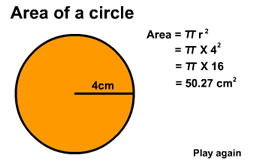# How to find the area of a circle

Want create site? Find Free WordPress Themes and plugins.

Circle is a shape in mathematics just like triangle, square, rectangle, oval etc. A circle is a sphere or a ball and it has many parts like the radius, sector, segment etc. Here we will first talk about the parts of a circle so that it is easy to find its area.

Related articles about How to find the area of a circle

How to find the area of a triangle

How to find circumference

How to find the area of a trapezoid

• Radius: It is simply just a line from the center to the wall of the circle whose name you will know as we move on.How to find the area of a circle

• Diameter: It is the double of the radius or the line that goes from one point on the circle through the center and connects to another point and it looks like a straight line. If the radius is 3 cm, the diameter will be 6cm.

• Circumference: It is just the wall of the circle or the part covering the circle and giving its shape like a ball. If it is big, the circle will have a big area and if it is small, the area of the circle will be small.

• Chord: It is simply any line on the circle that touches one part of the circumference to the other. The difference between this and the diameter is that it does not run through the center point of the circle.

• Arc: Arc is any part of the circumference.

• Tangent: It is a line that is touching the circumference and it is a straight line.

• Segment: It is a part that is cut off from the original circle.

• Sector: This is completely different from the segment while many people think they are similar. The sector is the part that is covered on one side by the arc and the other two lines are the radii.

To find the formula, you should know that you can make the circle into a rectangle by cutting into as small pieces as possible, therefore we get Area of circle =Area of rectangle

Area of rectangle is = base X height (base X radius)

The base is the same as radius of a circle and the height is half of the circumference of the circle. The circumference is equal to 2πr.

Base = half of circumference, ½ X 2πr

Cancel out the 2s and you will be left with πr.

Now, we know that base is πr and the height is radius,

Therefore, πr X r = πr²

This is the formula to find the area of any type of circle and here is an example. You have a circle with radius 7 inches, calculate the area.

Area = πr²

Area= (3.14) (7) ²

Area= (3.14) (49)

Area= 153.86 in²

In complicated problems, you may be given the diameter and you have to find out the radius. You need to divide the diameter by two.

Tips

• There is many more difficult formula about circle, that you will utilize later on, the formula is (x – h) ² + (y – k) ² = r² where the center is h and k.

• Check your math answers several times and make sure you use the correct value of π. If the question asks you to find it by 3.14159, use that.

Did you find apk for android? You can find new Free Android Games and apps.

We will be happy to hear your thoughts

This site uses Akismet to reduce spam. Learn how your comment data is processed.